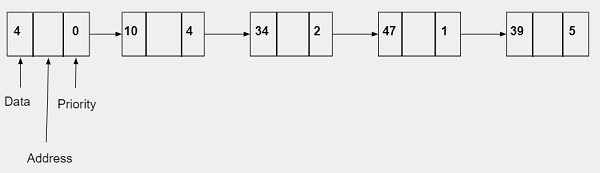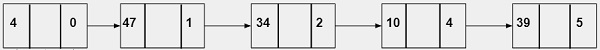# Priority Queue using Linked List in C

We are given with the data and the priority as an integer value and the task is to create a linked list as per the priority given and display the result.

Queue is a FIFO data structure in which the element which is inserted first is the first one to get removed. A Priority Queue is a type of queue in which elements can be inserted or deleted depending upon the priority. It can be implemented using queue, stack or linked list data structure. Priority queue is implemented by following these rules −

• Data or element with the highest priority will get executed before the data or element with the lowest priority.
• If two elements have the same priority than they will be executed in the sequence they are added in the list.

A node of a linked list for implementing priority queue will contain three parts −

• Data − It will store the integer value.
• Address − It will store the address of a next node
• Priority −It will store the priority which is an integer value. It can range from 0-10 where 0 represents the highest priority and 10 represents the lowest priority.

## Example

InputOutput## Algorithm

Start
Step 1-> Declare a struct node
Declare data, priority
Declare a struct node* next
Step 2-> In function Node* newNode(int d, int p)
Set Node* temp = (Node*)malloc(sizeof(Node))
Set temp->data = d
Set temp->priority = p
Set temp->next = NULL
Return temp
Step 3-> In function int peek(Node** head)
Step 4-> In function void pop(Node** head)
free(temp)
Step 5-> In function push(Node** head, int d, int p)
Set Node* temp = newNode(d, p)
Else
Loop While start->next != NULL && start->next->priority < p
Set start = start->next
Set temp->next = start->next
Set start->next = temp
Step 6-> In function int isEmpty(Node** head)
Step 7-> In function int main()
Set Node* pq = newNode(7, 1)
Call function push(&pq, 1, 2)
Call function push(&pq, 3, 3)
Call function push(&pq, 2, 0)
Loop While (!isEmpty(&pq))
Print the results obtained from peek(&pq)
Call function pop(&pq)
Stop

## Example

Live Demo

#include <stdio.h>
#include <stdlib.h>
// priority Node
typedef struct node {
int data;
int priority;
struct node* next;
} Node;
Node* newNode(int d, int p) {
Node* temp = (Node*)malloc(sizeof(Node));
temp->data = d;
temp->priority = p;
temp->next = NULL;
return temp;
}
}
free(temp);
}
void push(Node** head, int d, int p) {
Node* temp = newNode(d, p);
} else {
while (start->next != NULL &&
start->next->priority < p) {
start = start->next;
}
// Either at the ends of the list
// or at required position
temp->next = start->next;
start->next = temp;
}
}
// Function to check the queue is empty
}
// main function
int main() {
Node* pq = newNode(7, 1);
push(&pq, 1, 2);
push(&pq, 3, 3);
push(&pq, 2, 0);
while (!isEmpty(&pq)) {
printf("%d ", peek(&pq));
pop(&pq);
}
return 0;
}

## Output

2 7 1 3